> 文档中心 > 详解时间复杂度和空间复杂度

# 详解时间复杂度和空间复杂度### 文章目录

• 1、算法效率
• 2、时间复杂度
• 2.1、 时间复杂度的定义
• 2.2 、大O的渐进表示法
• 2.2.1 、O(1) 时间复杂度示例
• 2.2.2、O(N) 时间复杂度示例
• 2.2.3、M+N时间复杂度示例
• 2.2.4、冒泡排序时间复杂度O(N^2^)
• 2.2.5、strstr 函数时间复杂度O(N)
• 2.2.6、二分查找时间复杂度O(log2^N^)
• 2.2.7、递归时间复杂度
• 2.2.8、递归里面还包含有其他的时间复杂度
• 2.2.9、斐波那契数列的时间复杂度
• 3、空间复杂度
• 3.1、空间复杂度
• 3.1.1、冒泡排序的空间复杂度
• 3.1.2、斐波那契数列的空间复杂度
• 3.1.3、阶乘递归Fac的空间复杂度
• 3.1.4、斐波那契递归Fib的空间复杂度
• 4、常见的复杂度对比
• 总结

# 2、时间复杂度

## 2.1、 时间复杂度的定义``// 请计算一下Func1中++count语句总共执行了多少次？void Func1(int N){int count = 0;for (int i = 0; i < N ; ++ i){for (int j = 0; j < N ; ++ j){++count;}}for (int k = 0; k < 2 * N ; ++ k){++count;}int M = 10;while (M--){++count;}printf("%d\n", count);}``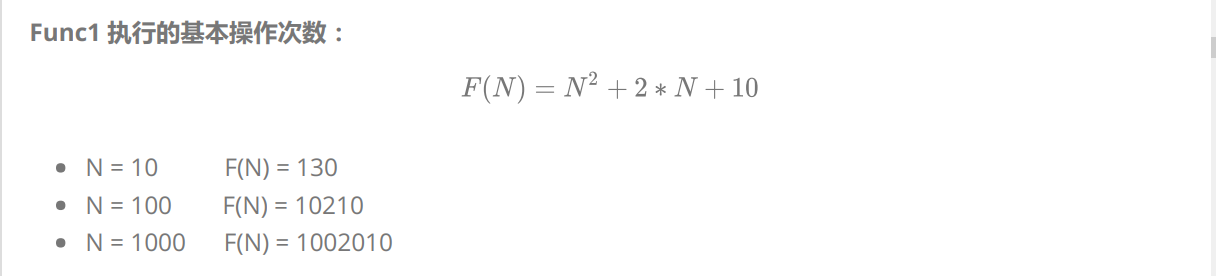❓那Func1的时间复杂度就是 N 2+2 * N + 10 吗？

💯 回答：当然不是啦！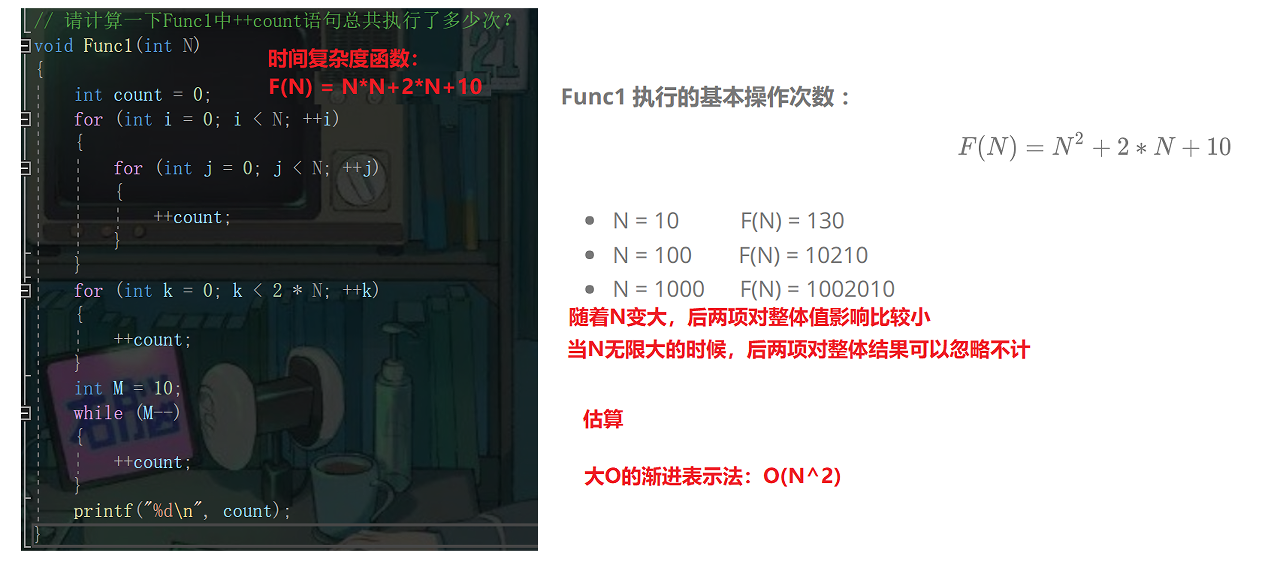## 2.2 、大O的渐进表示法

1、用常数1取代运行时间中的所有加法常数。(只有常数就会用1去取代 ）
2、在修改后的运行次数函数中，只保留最高阶项。（忽略那些对结果影响不大的项）
3、如果最高阶项存在且不是1，则去除与这个项目相乘的常数。得到的结果就是大O阶。

### 2.2.1 、O(1) 时间复杂度示例

``// 计算Func4的时间复杂度？void Func4(int N){int count = 0;for (int k = 0; k < 100; ++ k){++count;}printf("%d\n", count);}``

Func4 这段代码我们可以看到，里面就一层for循环，而且循环次数是常数次，更准确点是100次，但是我们上面说了不需要计算到准确的次数，那么这里的常数次无论是多大，只要是常数，就根据推导大O阶方法 1 知：O(1) 复杂度类型。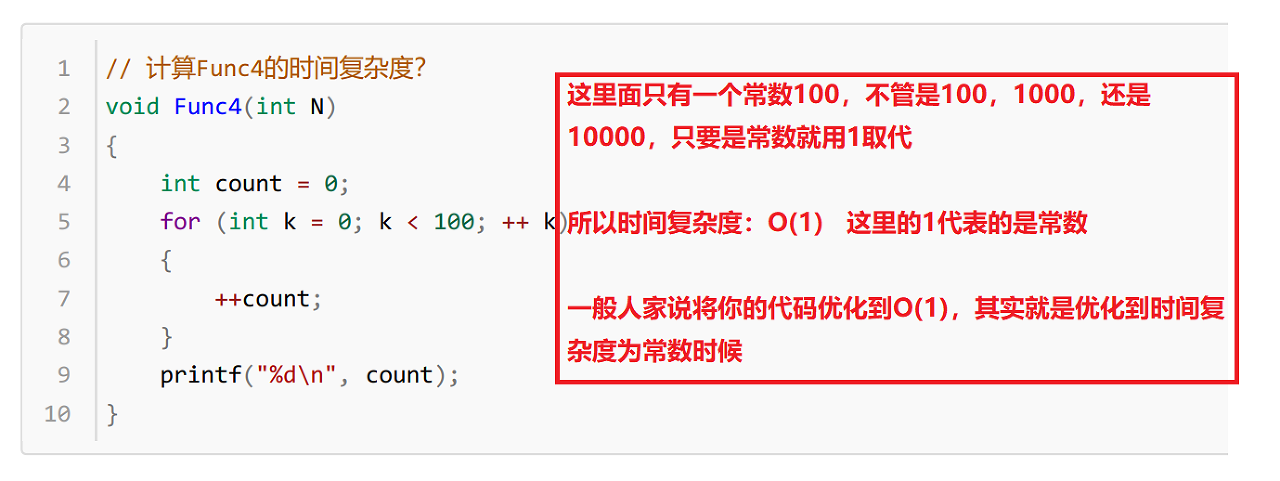### 2.2.2、O(N) 时间复杂度示例

``// 计算Func2的时间复杂度？void Func2(int N){    int count = 0;    for (int k = 0; k < 2 * N ; ++ k)    { ++count;    }    int M = 10;    while (M--)    { ++count;    }    printf("%d\n", count);}``

Func2在这里我们不难看出其代码中存在的数学函数表达式为：F(N) = 2 * N + 10，这里根据推导大O阶方法二和三可以得出，Func2的时间复杂度为：O(N)。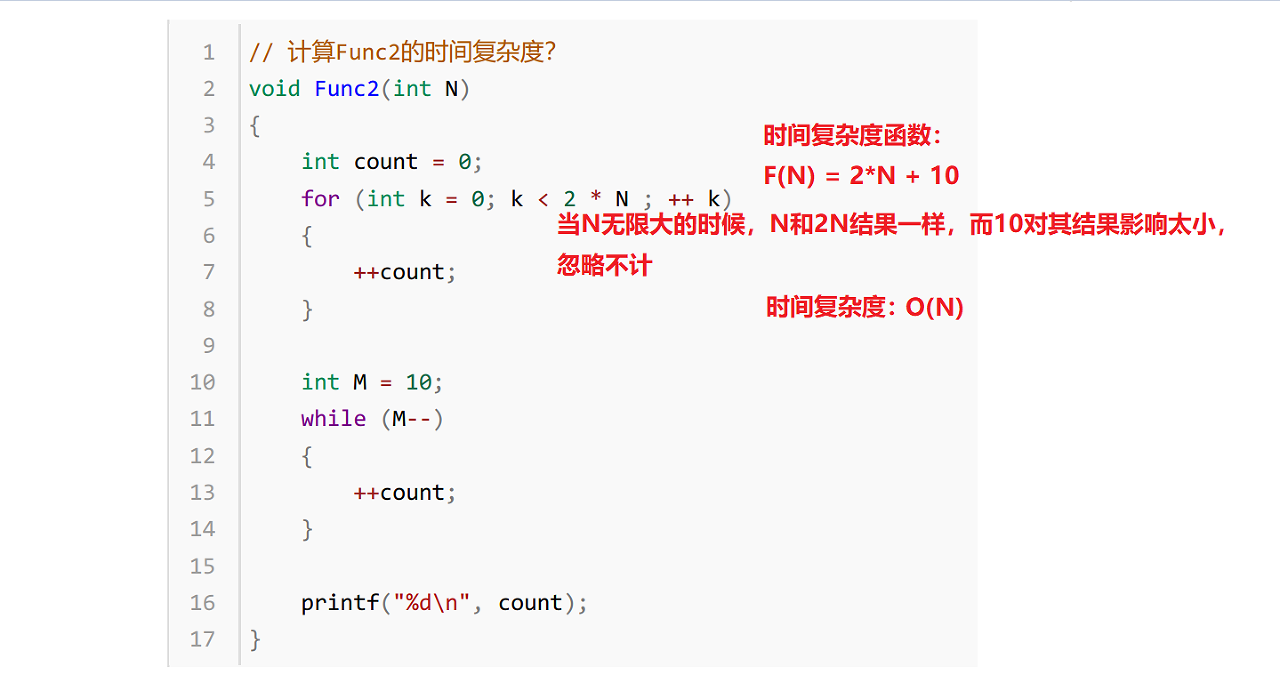### 2.2.3、M+N时间复杂度示例

``// 计算Func3的时间复杂度？void Func3(int N, int M){    int count = 0;    for (int k = 0; k < M; ++ k)    { ++count;    }    for (int k = 0; k < N ; ++ k)    { ++count;    }    printf("%d\n", count);}``

Func3可以看出是有两个for循环而循环的次数都是未知数，所以这里时间复杂度为：O(M+N)；当然这里依然存在三种情况考虑一下：当M>>N时，就是O(M)；当M<<N时，这里就是O(N)，当两者相似时，就是2M/2N，即O(M)/O(N)。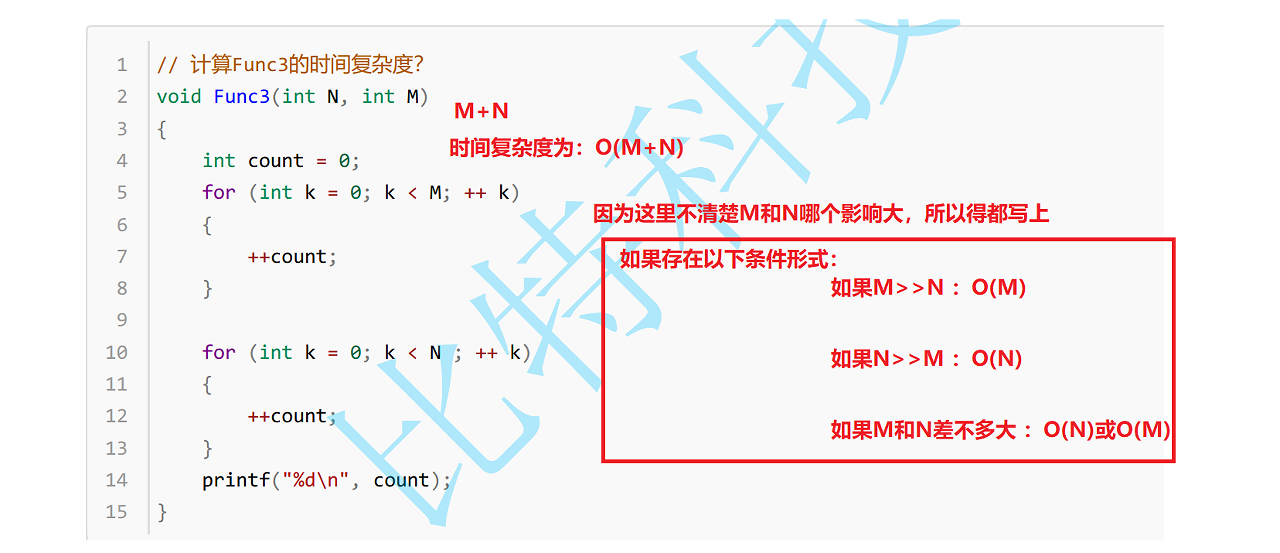### 2.2.4、冒泡排序时间复杂度O(N2)

``/ 计算BubbleSort的时间复杂度？void BubbleSort(int* a, int n){    assert(a);    for (size_t end = n; end > 0; --end)    {    int exchange = 0;    for (size_t i = 1; i < end; ++i)    {    if (a[i-1] > a[i])    {    Swap(&a[i-1], &a[i]);    exchange = 1;    }    }    if (exchange == 0)   break;   }}``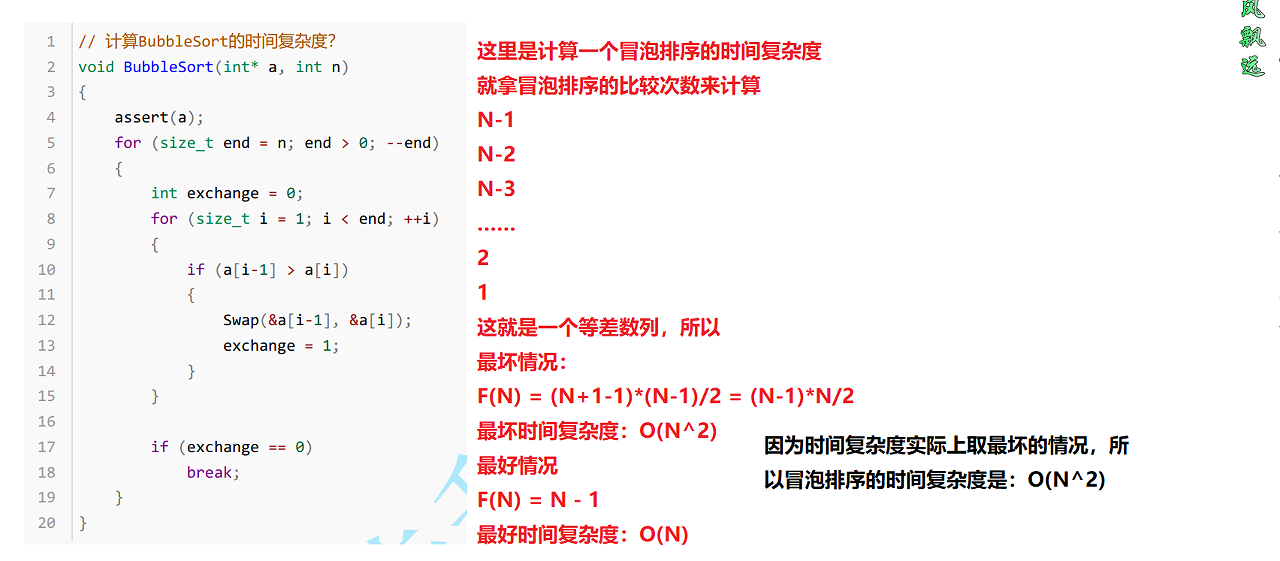### 2.2.5、strstr 函数时间复杂度O(N)

``// 计算strchr的时间复杂度？const char * strchr ( const char * str, int character );``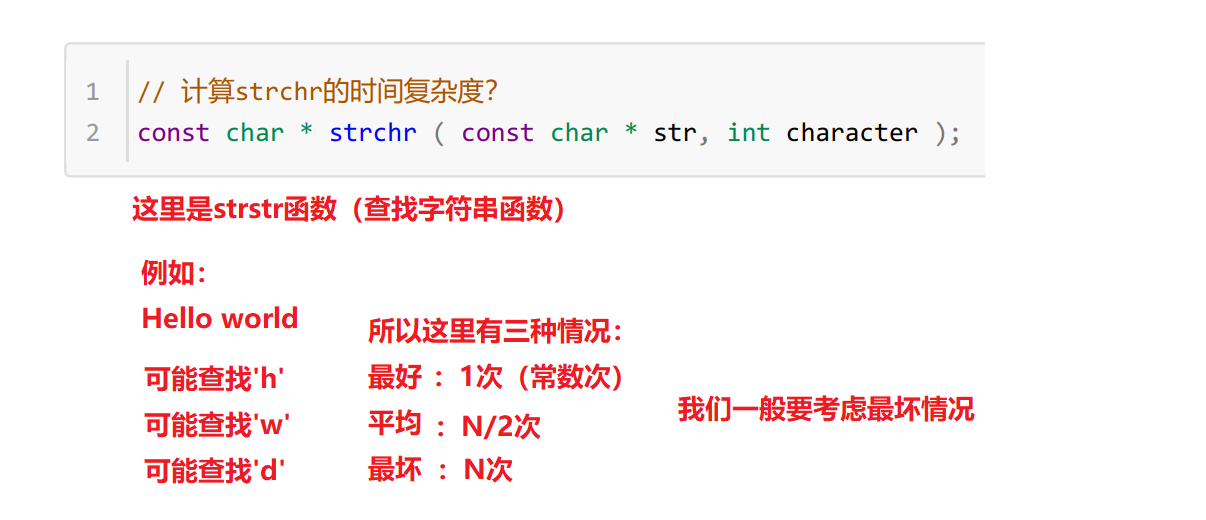### 2.2.6、二分查找时间复杂度O(log2N)

``// 计算BinarySearch的时间复杂度？int BinarySearch(int* a, int n, int x){    assert(a);    int begin = 0;    int end = n-1;    while (begin < end)    { int mid = begin + ((end-begin)>>1); if (a[mid] < x)     begin = mid+1; else if (a[mid] > x)     end = mid; else     return mid;    }    return -1;}``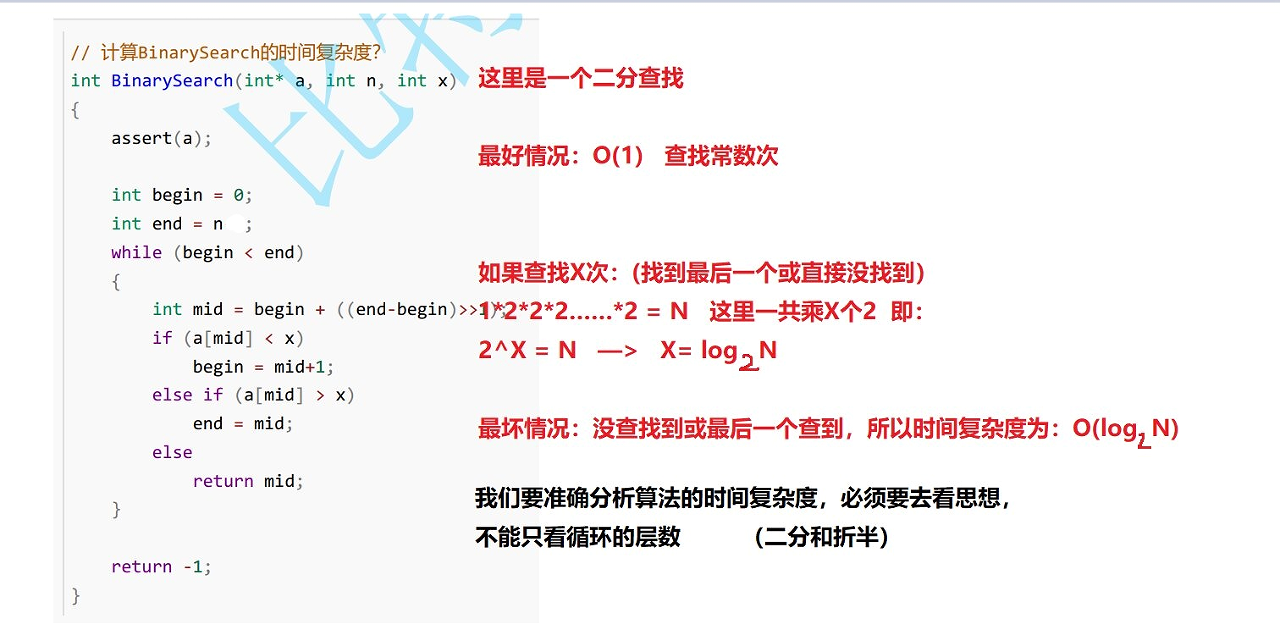（这里单纯的讨论二分查找这个思想，不考虑二分查找需要有序的数组，有序是二分查找需要付出的代价）

N     O(N)   log(2)N
1000   1000    10
100W    100W    20
10亿   10亿    30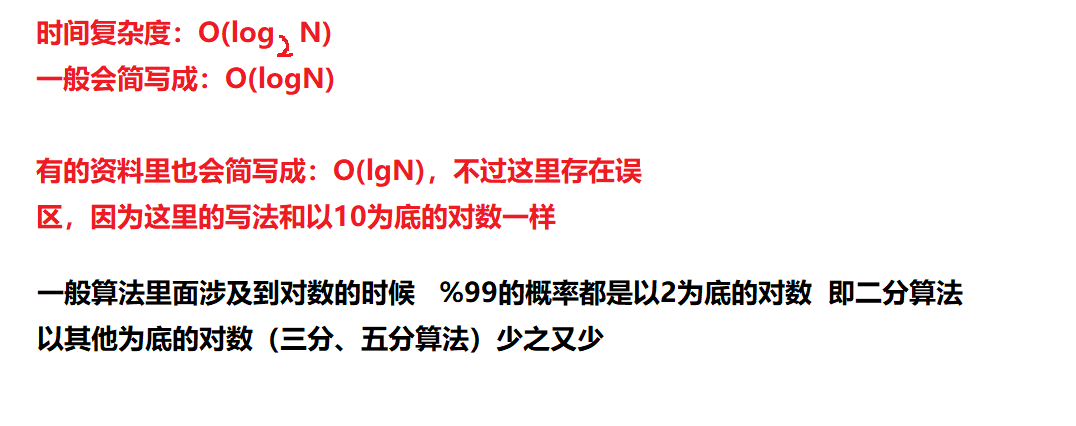### 2.2.7、递归时间复杂度

``// 计算阶乘递归Fac的时间复杂度？long long Fac(size_t N){if(0 == N)return 1;return Fac(N-1)*N;}``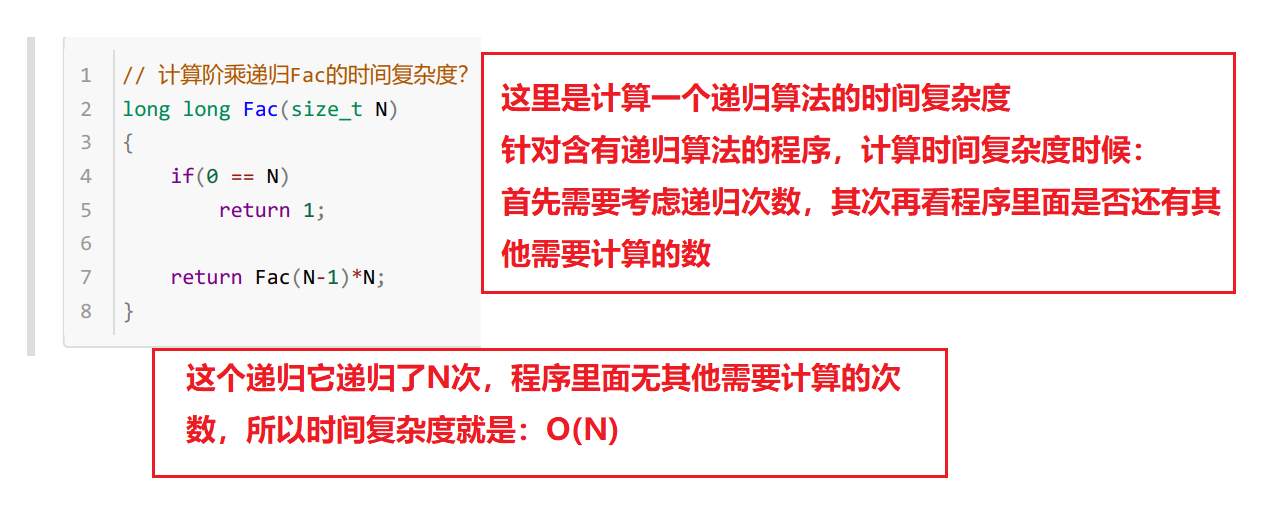### 2.2.8、递归里面还包含有其他的时间复杂度

``// 计算阶乘递归Fac的时间复杂度？long long Fac(size_t N){    if (0 == N)return 1;    for (int i = 0; i < N; i++)    {    printf("%d ", i);    }printf("\n");return Fac(N - 1) * N;}``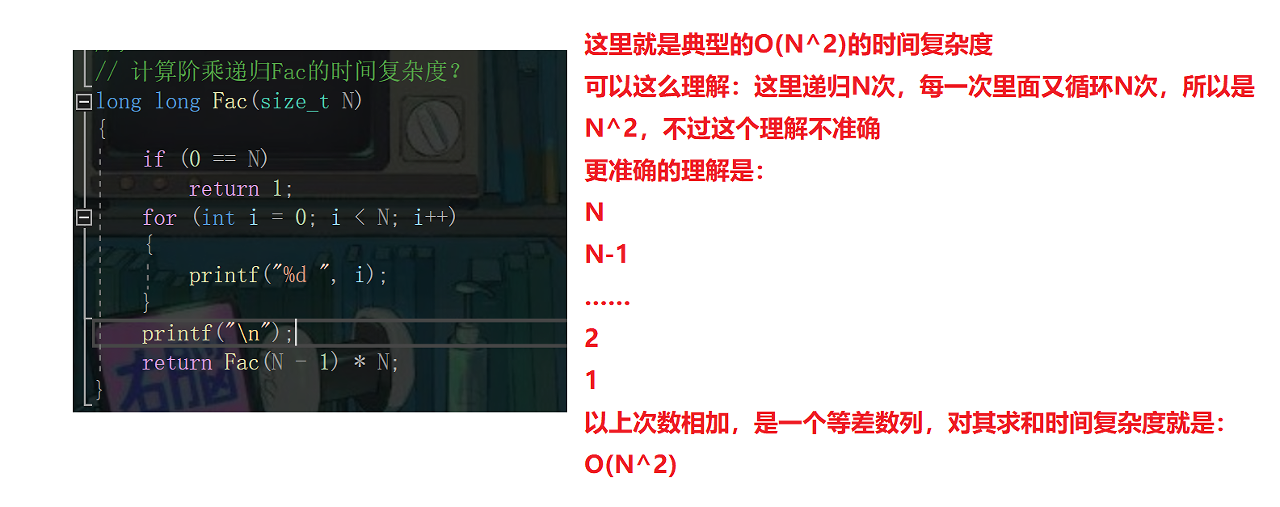1、如果每次函数调用次数是O(1)，那就看他的递归次数；
2、如果每次函数调用次数不是O(1)，那就看他的递归调用次数中的累加；

### 2.2.9、斐波那契数列的时间复杂度

``// 计算斐波那契递归Fib的时间复杂度？long long Fib(size_t N){    if(N < 3)    return 1;    return Fib(N-1) + Fib(N-2);}``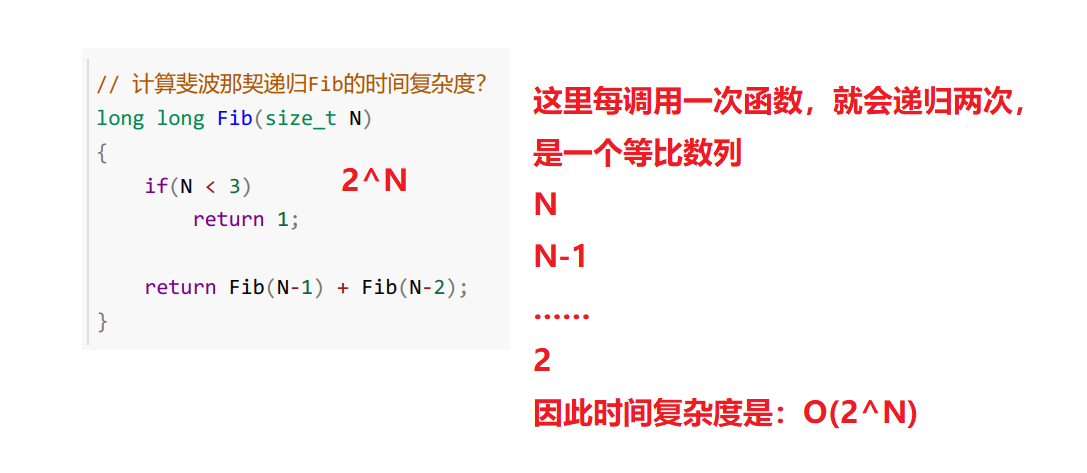# 3、空间复杂度

## 3.1、空间复杂度

(注意：时间复杂度看次数，空间复杂度看变量的个数）

### 3.1.1、冒泡排序的空间复杂度

``// 计算BubbleSort的空间复杂度？void BubbleSort(int* a, int n){    assert(a);    for (size_t end = n; end > 0; --end)    { int exchange = 0; for (size_t i = 1; i < end; ++i) {     if (a[i-1] > a[i])     {  Swap(&a[i-1], &a[i]);  exchange = 1;     } } if (exchange == 0)     break;    }}``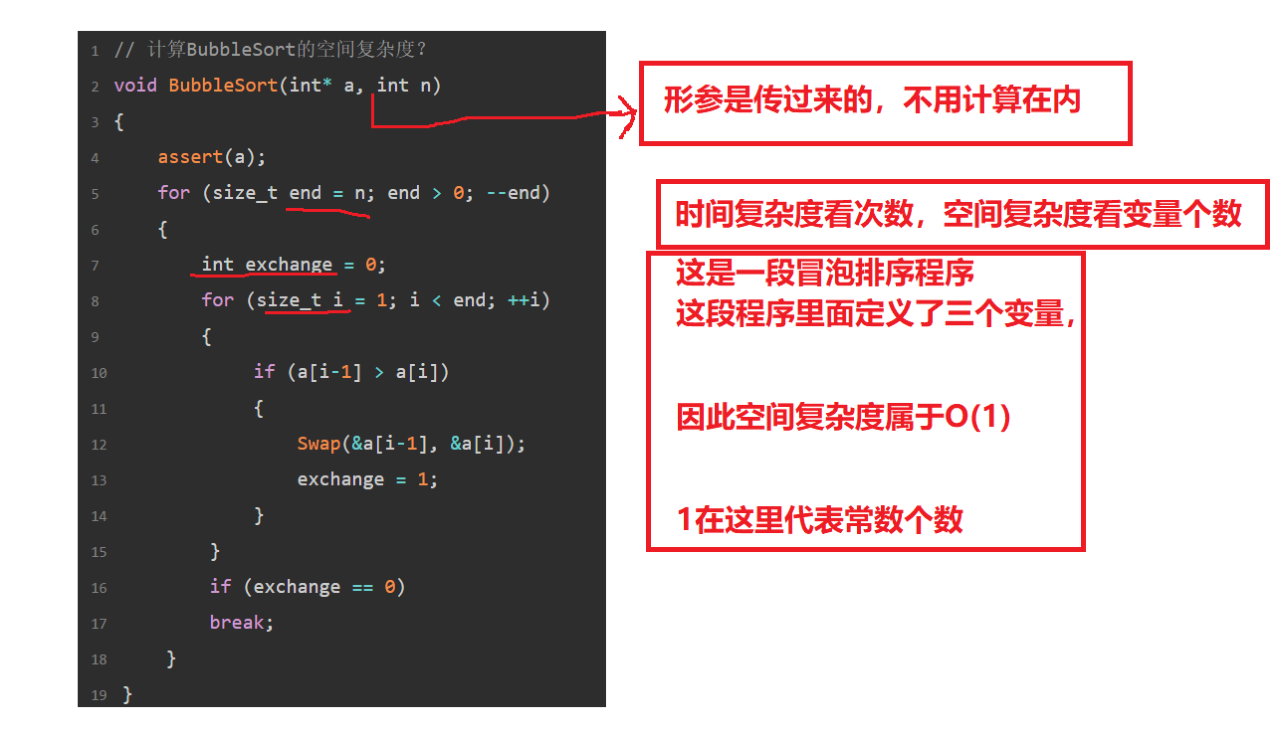### 3.1.2、斐波那契数列的空间复杂度

``// 计算Fibonacci的空间复杂度？// 返回斐波那契数列的前n项long long* Fibonacci(size_t n){    if(n==0) return NULL;    long long * fibArray = (long long *)malloc((n+1) * sizeof(long long));    fibArray = 0;    fibArray = 1;    for (int i = 2; i <= n ; ++i)    { fibArray[i] = fibArray[i - 1] + fibArray [i - 2];    }    return fibArray;}``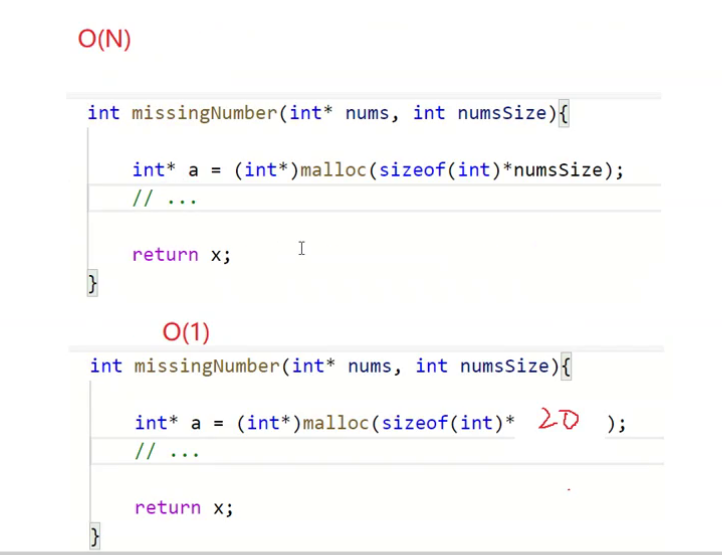### 3.1.3、阶乘递归Fac的空间复杂度

``// 计算阶乘递归Fac的空间复杂度？long long Fac(size_t N){    if(N == 0) return 1;    return Fac(N-1)*N;}``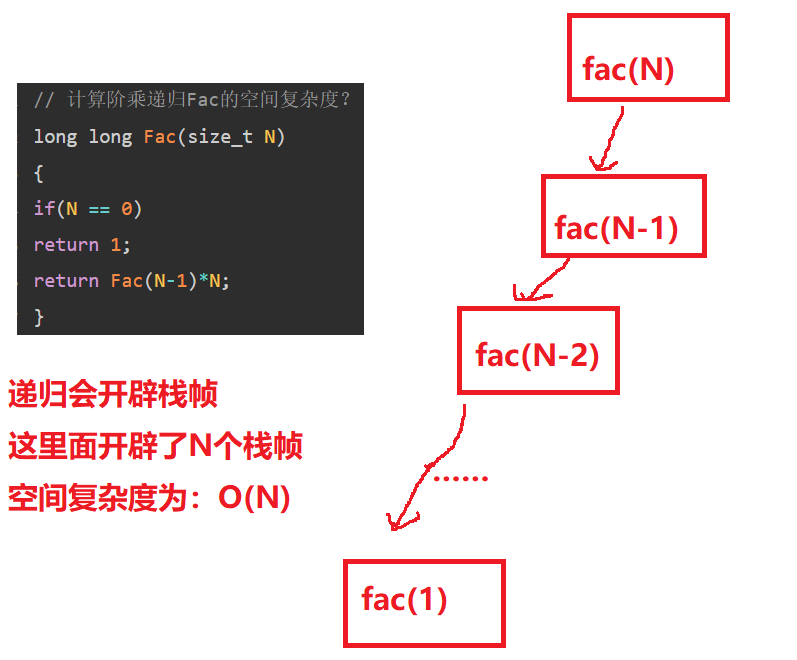### 3.1.4、斐波那契递归Fib的空间复杂度

``// 计算斐波那契递归Fib的空间复杂度？long long Fib(size_t N){    if(N < 3) return 1;    return Fib(N-1) + Fib(N-2);}``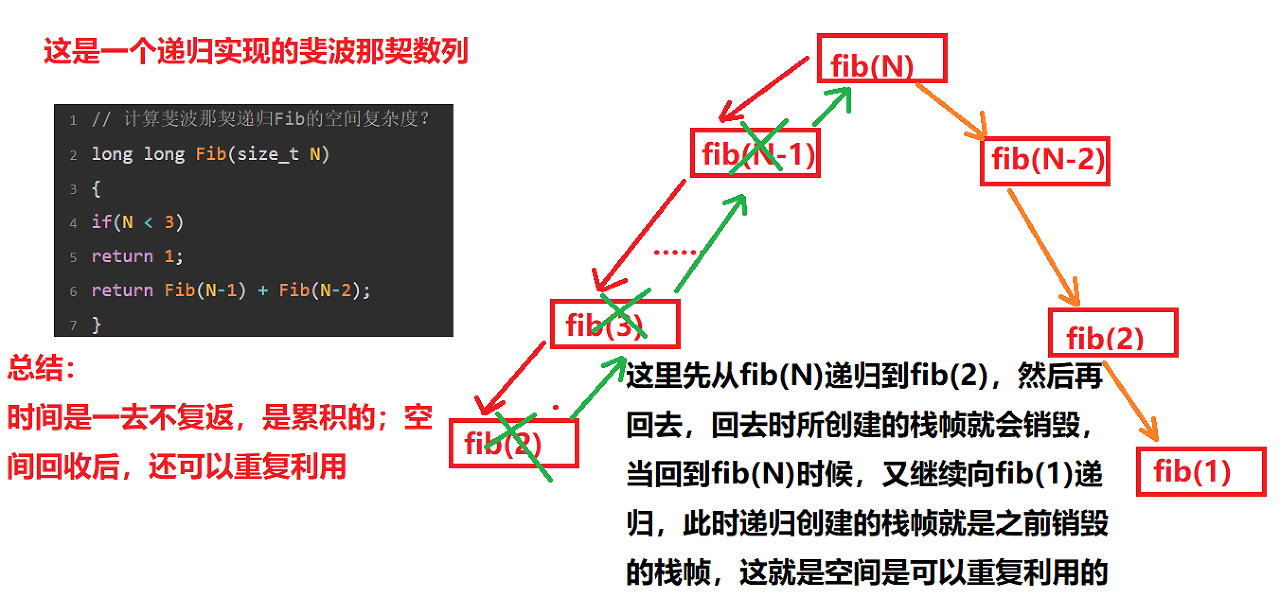# 4、常见的复杂度对比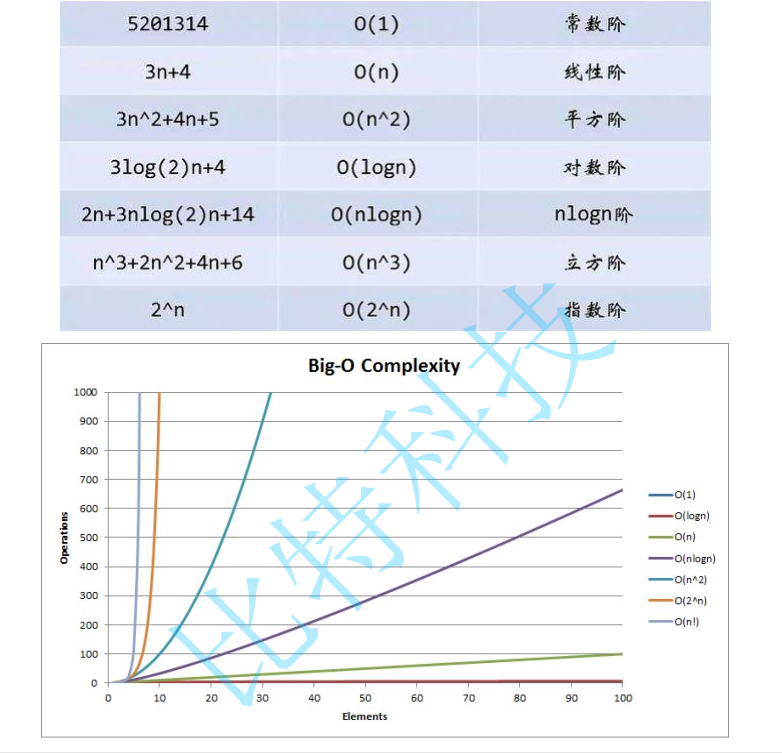# 总结